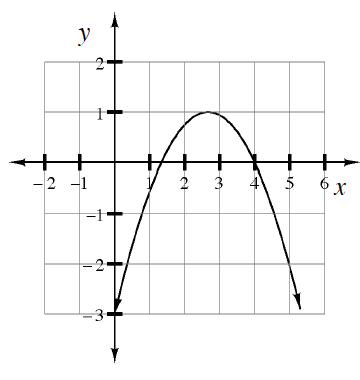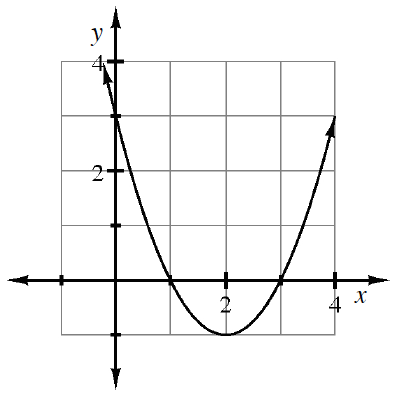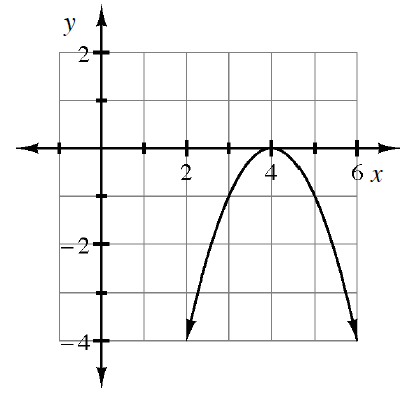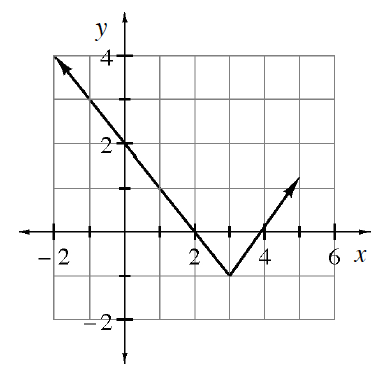### Home > CCAA8 > Chapter 5 Unit 6 > Lesson CCA: 5.1.2 > Problem5-25

5-25.

Determine the domain and range of each of the following graphs.

1.• To determine the range, locate the vertex of the parabola.

• Domain: all numbers
Range: $y\le1$

1.• See part (a).

1.• See part (a).

1.• Domain: all numbers
Range: $y\ge−1$